# MA.4.DP.1.1Export Print
Collect and represent numerical data, including fractional values, using tables, stem-and-leaf plots or line plots.

### Examples

A softball team is measuring their hat size. Each player measures the distance around their head to the nearest half inch. The data is collected and represented on a line plot.

### Clarifications

Clarification 1: Denominators are limited to 2, 3, 4, 5, 6, 8, 10, 12, 16 and 100.
General Information
Subject Area: Mathematics (B.E.S.T.)
Strand: Data Analysis and Probability
Status: State Board Approved

## Benchmark Instructional Guide

### Terms from the K-12 Glossary

• Line Plot
• Stem-and-Leaf Plot

### Vertical Alignment

Previous Benchmarks

Next Benchmarks

### Purpose and Instructional Strategies

The purpose of this benchmark is to collect authentic data and display the data using the appropriate format. This concept builds on collecting and displaying whole number data using line plots, bar graphs, and tables in grade 3 (MA.3.DP.1.1). Student data in grade 4 will be displayed using stem-and-leaf plots, in addition to other methods. In grade 5, fractional and decimal data will be included (MA.5.DP.1.1).
• A stem-and-leaf plot displays numerical data and use place value to display data frequencies. In a stem-and-leaf-plot, a number is decomposed so that leaves represent the smallest part of a number (e.g., ones, fraction less than 1) and the stem consists of all its other place values (e.g., hundreds, tens, ones in fractions greater than 1). Stem-and- leaf plots help students build line plots. Stem-and-leaf plots can help students identify benchmarks for their number lines when creating a line plot.
• During instruction connections should be made between how data is represented on stem-and-leaf and line plots. Stem-and-leaf plots can help students identify benchmarks for their number lines when creating a line plot.
• A stem-and-leaf plot organizes data by size (e.g., least to greatest or greatest to least) and identifies the mode of a data set as the stem with the greatest number of leaves. It can be used to find the median and range of the data set.
• Measurement data can be gathered (including measuring with precision to the nearest $\frac{\text{1}}{\text{16}}$ inch) and displayed on tables, line plots, and stem and leaf plots. The data is the same for each of the displays below.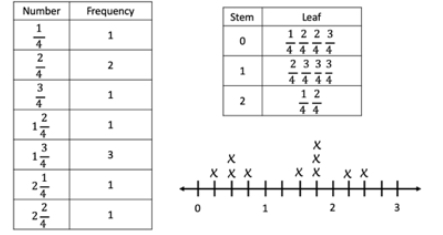• Instruction of line plots should first focus on creating appropriate number lines that allow a data set to be displayed.

### Common Misconceptions or Errors

• For line plots, students may misread a number line and have difficulty because they use whole-number names when counting fractional parts on a number line instead of the fraction name. Students also count the tick marks on the number line to determine the fraction, rather than looking at the “distance” or “space” between the marks.
• For stem-and-leaf plots, students may read they key incorrectly. Some students may try to represent numerical data in a stem-and-leaf plot without first arranging the leaves for each stem in order.

### Strategies to Support Tiered Instruction

• Instruction includes opportunities to read number lines with fraction values and opportunities for students to use concrete models and drawing of number lines to connect their learning with fraction understanding.
• For example, students plot fourths on the number line, paying particular attention to what each tick mark and the “distance” between each tick mark represents.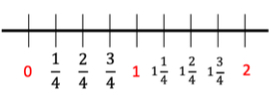• For example, utilizing fraction strips or tiles, students connect fractional parts to the measurement on a number line.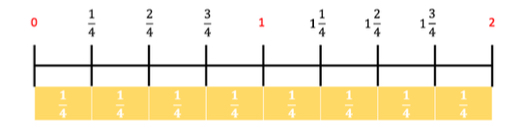• Instruction includes representing numerical data in a stem and leaf plot and ordering the data from least to greatest. The stem will be the greatest place value of the largest number in the set. With a set of mixed numbers, the stems will be the whole numbers.
• For example, create a stem and leaf plot using the data set shown.

Data set: 6, 8, 11, 20, 24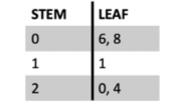• Instruction includes representing numerical data in a stem-and-leaf plot and writing the data set on index cards or sticky notes.
• Example: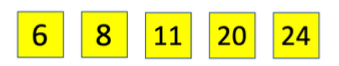• After organizing the data set in order from least to greatest, the students rip each number, separating the place values and place them on the graphic organizer. The greater place value will be the stem, the tens place for this example. The lesser place value will be the leaf, the ones place in this example. Since the stems will only be labeled once, the numbers with the same place value will be stacked on top of each other. Each of the leaves will be represented, even if repeated. Numbers with 0 in the tens place will be represented by a 0 for the stem.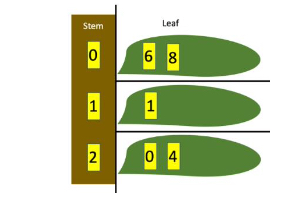Measure the length of 10 used pencils in the class to the nearest   inch. Create a stem-and-leaf plot and a line plot to represent the lengths of all ten pencils.

### Instructional Items

Instructional Item 1

Laura was given the data in the chart below.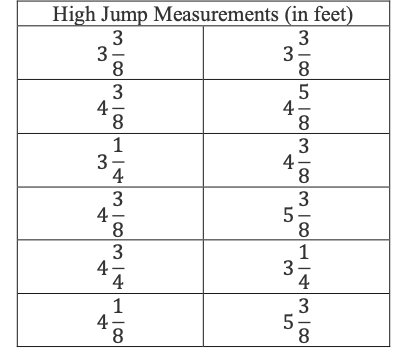She was asked to create a line plot to represent her data. How many X’s will she place above 4
• a. 3
• b. 4
• c. 8
• d. 12

*The strategies, tasks and items included in the B1G-M are examples and should not be considered comprehensive.

## Related Courses

This benchmark is part of these courses.
5012060: Mathematics - Grade Four (Specifically in versions: 2014 - 2015, 2015 - 2022, 2022 and beyond (current))
7712050: Access Mathematics Grade 4 (Specifically in versions: 2014 - 2015, 2015 - 2018, 2018 - 2022, 2022 and beyond (current))
5012065: Grade 4 Accelerated Mathematics (Specifically in versions: 2019 - 2022, 2022 and beyond (current))
5012015: Foundational Skills in Mathematics 3-5 (Specifically in versions: 2019 - 2022, 2022 and beyond (current))

## Related Access Points

Alternate version of this benchmark for students with significant cognitive disabilities.
MA.4.DP.1.AP.1: Sort and represent numerical data, including fractional values using tables or line plots (when given a scaled number line). Data set to include only whole numbers and halves.

## Related Resources

Vetted resources educators can use to teach the concepts and skills in this benchmark.

## Formative Assessments

Science Experiment Part One:

Students are asked to use a given set of data to create a line plot with an appropriate scale.

Type: Formative Assessment

Bulk Candy Part One:

Students are asked to use a given set of data to create a line plot with an appropriate scale.

Type: Formative Assessment

Race Cars Part One:

Students are asked to use a given set of data to create a line plot with an appropriate scale.

Type: Formative Assessment

Rock Measurements Part One:

Students are asked to use a given set of data to create a line plot with an appropriate scale.

Type: Formative Assessment

## Lesson Plans

Motion for Speed:

Students will investigate the relationships between the initial speed of a car and the distance required for it to stop.  Students will record their results onto a student guide to determine how a car moving faster or slower affects its ability to stop.  Finally, students will investigate why we have different speed limits on different roads in this integrated lesson plan.

Type: Lesson Plan

One Leg Up:

In this lesson the students will be using a line plot to display a data set of measurements in fractions of a unit (1/2, 1/4, 1/8). They will be measuring different lengths of insects, recording the lengths, and then plotting the data on a line plot. From this data they will solve problems involving the addition and subtraction of fractions.

Type: Lesson Plan

Measure Up! Measuring to make a line plot.:

In this lesson, students will generate measurement data by measuring lengths using rulers and show the data by making a line plot.

Type: Lesson Plan

Fourth graders will help Cookies and Treats find cost-effective and eco-friendly packaging for its cookies. Students will organize data and compare prices using decimal notation in order to develop a procedure for choosing packaging for cookies.  Students will use multiplication and division of whole numbers to plan for how many packages to order.

Type: Lesson Plan

Marshmallow Math:

In this lesson, students are physically engaged in measuring distances of tossed marshmallows to the nearest 1/2 foot. Using their measurements, they will represent the data on a line plot and then solve word problems involving addition and subtraction of mixed numbers. This is a fun lesson that motivates students to become excited about the difficult world of fractions.

Type: Lesson Plan

## Original Student Tutorials

Join us as Breanna learns to use a line plot to examine measurement data she needs to create bracelets for her friends, in this interactive tutorial.

Type: Original Student Tutorial

Logging Lengths with Line Plots:

Learn how to create a line plot and analyze data in the line plot in this interactive tutorial.  You will also see how to add and subtract using the line plot to solve problems based on the line plots.

Type: Original Student Tutorial

## Perspectives Video: Teaching Idea

Using Manipulatives to Create Stem and Leaf Plots:

Unlock an effective teaching strategy for teaching stem and leaf plots in this Teacher Perspectives video for educators.

Type: Perspectives Video: Teaching Idea

## Teaching Idea

Ball Bounce Experiment:

Students investigate different balls' abilities to bounce and represent the data they collect graphically.

Type: Teaching Idea

## Virtual Manipulative

KidsZone: Create a Graph:

Create bar, line, pie, area, and xy graphs.

Type: Virtual Manipulative

## STEM Lessons - Model Eliciting Activity

Fourth graders will help Cookies and Treats find cost-effective and eco-friendly packaging for its cookies. Students will organize data and compare prices using decimal notation in order to develop a procedure for choosing packaging for cookies.  Students will use multiplication and division of whole numbers to plan for how many packages to order.

## MFAS Formative Assessments

Bulk Candy Part One:

Students are asked to use a given set of data to create a line plot with an appropriate scale.

Race Cars Part One:

Students are asked to use a given set of data to create a line plot with an appropriate scale.

Rock Measurements Part One:

Students are asked to use a given set of data to create a line plot with an appropriate scale.

Science Experiment Part One:

Students are asked to use a given set of data to create a line plot with an appropriate scale.

## Original Student Tutorials Mathematics - Grades K-5

Join us as Breanna learns to use a line plot to examine measurement data she needs to create bracelets for her friends, in this interactive tutorial.

Logging Lengths with Line Plots:

Learn how to create a line plot and analyze data in the line plot in this interactive tutorial.  You will also see how to add and subtract using the line plot to solve problems based on the line plots.

## Student Resources

Vetted resources students can use to learn the concepts and skills in this benchmark.

## Original Student Tutorials

Join us as Breanna learns to use a line plot to examine measurement data she needs to create bracelets for her friends, in this interactive tutorial.

Type: Original Student Tutorial

Logging Lengths with Line Plots:

Learn how to create a line plot and analyze data in the line plot in this interactive tutorial.  You will also see how to add and subtract using the line plot to solve problems based on the line plots.

Type: Original Student Tutorial

## Parent Resources

Vetted resources caregivers can use to help students learn the concepts and skills in this benchmark.